# Metric and projective geometry : Theorem of Thales

One of the major theorems metric geometry is the statement by Thales of Miletus. Together with Pythagorean theorem establish the fundamentals of axiomatic metric and projective geometries.

Thales of Miletus (Greek Θαλῆς ὁ Μιλήσιος) (ca. 630 – 545 to. C ) was the initiator of rational inquiry about the universe. He is considered the first philosopher in the history of Western philosophy, and was the founder of the Ionian school of philosophy, according to the testimony of Aristotle. It was the first and most famous of the Seven Sages of Greece (the learned astronomer), and would have, according to an ancient tradition not sure, disciple and protected as Pythagoras.

It was also one of the greatest mathematicians of his time, focusing its main contributions in the foundations of geometry.(W)

## Statement of the First Theorem of Thales

The Thales theorem sets notion of similarity between two triangles relating the length of two sides. Set a projective invariant applies to cylindrical projection systems: The simple reason.

If any two lines cut by several parallel lines,corresponding segments are proportional both,namely, correspond to equal ,in addition and subtraction in.

If a triangle is a line parallel to either side, you get two triangles similar.(W)

The following theorem states the equality between the ratios of two corresponding sides of two similar triangles:

• m / n = m '/ n'
• m / n = (m m ')/(n n ')
• n / p = (n n ')/p '

### Applications: Scales

The notion of similarity is associated with the scale. Two similar forms (Likewise but different size) only vary in scale representation.

The scale is the mathematical relationship between the actual dimensions of the drawing and actually represents a plane or map.(W)

Scale = Linear Measure in Drawing / linear measurement of the real

E = D / R

For example, Scale E = 3/4 indicates that 4 units of measurement of the real, we 3 in the drawing.

#### Elements that form a graphic scale.

A scale built on a rectilinear support. Each numbered part is called module. The part that is left of zero is called contraescala.

#### Construction of Scales

As an application example suppose we want to build the scale 7/9.

Holder will use a long rectilinear 7 units represent measurements and an auxiliary line drawing of nine units length attached at one end to the above, which represents the measure of reality.

Unite the two free ends of both lines and go tracing straight parallel to the latter for each of the units of the auxiliary line.

## Training

The following exercises can drill and anchor the concepts discussed that will be critical to, later, understand that we will use in projective invariant representation systems.

1-.Dividing a segment s = AB proportional parts others, b, c .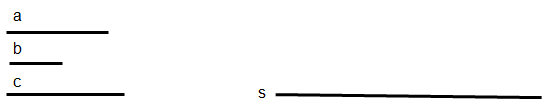2-.If a / b = c / x, find the segment x ,fourth proportional three segments, b, c given.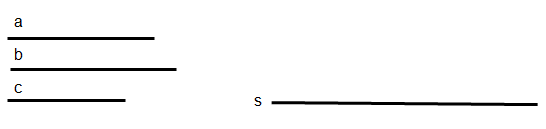3-.If a / b = b / x. Find the segment x ,two third segments proportional to, b given.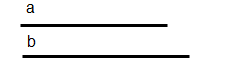4-.Find two segments x and y, known their sum s and difference d.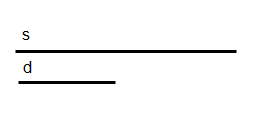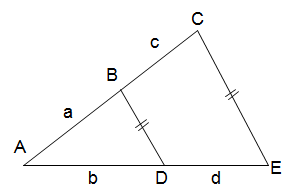5-.In the figure below is fulfilled:

Indicate whether the relation is true (V..) or false (F) in each case

• V F AD . AE = AB . BC
• V F AD / BC = AB / DE
• V F AB . DE = AD . BC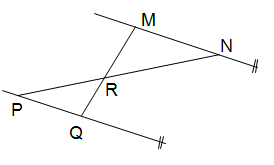6-.In the figure below is fulfilled:

Indicate whether the relation is true (V..) or false (F) in each case

• V F MN / NR = QP . QR
• V F MN . QR = MR . QP
• V F PR / RN = QR / RM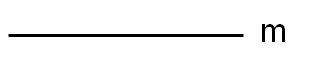7.- Given a segment m, determine two segments p and q knowing that:

• m = q + p
• p/q =2/38.- Given a segment m, determine two segments p and q knowing that:

• m = q – p
• p/q =2/3

Metric geometry